An inventory model of instantaneous deteriorating items with controllable deterioration rate for time dependent demand and holding costAn inventory model of instantaneous deteriorating items
with controllable deterioration rate for time dependent demand
and holding cost

Vinod Kumar Mishra

Department of Computer Science & Engineering, B. T. Kumaon Institute of Technology (India)

Accepted: March 2013

Mishra, V.K. (2013). An inventory model of instantaneous deteriorating items with controllable deterioration rate for time dependent demand and holding cost. Journal of Industrial Engineering and Management, 6(2), 495-506. http://dx.doi.org/10.3926/jiem.530

---------------------

Abstract:

Purpose: The purpose of this paper to develop an inventory model for instantaneous deteriorating items with the consideration of the facts that the deterioration rate can be controlled by using the preservation technology (PT) and the holding cost & demand rate both are linear function of time which was treated as constant in most of the deteriorating inventory model.

Design/methodology/approach: Developed the mathematical equation of deterministic deteriorating inventory model in which demand rate and holding cost both is linear function of time, deterioration rate is constant, backlogging rate is variable and depend on the length of the next replenishment, shortages are allowed and partially backlogged and obtain an analytical solution which optimizes the total cost of the proposed inventory model.

Findings: The model can be applied for optimizing the total inventory cost of deteriorating items inventory for such business enterprises where they use the preservation technology to control the deterioration rate under other assumptions of the model.

Originality/value: The inventory system for deteriorating items has been an object of study for a long time, but little is known about the effect of investing in reducing the rate of product deterioration and their significant impact in the business. The proposed model is effective as well as efficient for the business organization that uses the preservation technology to reduce the deterioration rate of the instantaneous deteriorating items of the inventory.

Keywords: inventory, deteriorating items, shortages, controllable deterioration rate, partial backlogging, preservation technology, time dependent holding cost

---------------------

1. Introduction

Inventory System is one of the main stream of the Operations Research which is essential in business enterprises and Industries. Interest in the subject is constantly increasing, and its development in recent years closely parallels the development of operations research in general. Some authors even claim that “More operations research has been directed towards inventory control than toward any other problem area in business and industry” and among these the deteriorating items inventory have gain large emphasis in last decade. The inventory system for deteriorating items has been an object of study for a long time, but little is known about the effect of investing in reducing the rate of product deterioration. So in this paper, an inventory model is developed to consider the fact that the uses of preservation technology reduce the deterioration rate by which the retailer can reduce the economic losses, improve the customer service level and increase business competitiveness.

Inventory of deteriorating items first studied by Within (1957), he considered the deterioration of fashion goods at the end of prescribed storage period. Ghare and Schrader (1963) extended the classical EOQ formula with exponential decay of inventory due to deterioration and gave a mathematical model of inventory of deteriorating items. Dave and Patel (1981) developed the first deteriorating inventory model with linear trend in demand. He considered demand as a linear function of time. Goyal and Giri (2001) gave recent trends of modeling in deteriorating items inventory. They classified inventory models on the basis of demand variations and various other conditions or constraints. Ouyang, Wu and Cheng (2005) developed an inventory model for deteriorating items with exponential declining demand and partial backlogging. Alamri and Balkhi (2007) studied the effects of learning and forgetting on the optimal production lot size for deteriorating items with time varying demand and deterioration rates. Dye and Ouyang (2007) found an optimal selling price and lot size with a varying rate of deterioration and exponential partial backlogging. They assume that a fraction of customers who backlog their orders increases exponentially as the waiting time for the next replenishment decreases.

In (2008) Roy Ajanta developed a deterministic inventory model when the deterioration rate is time proportional, demand rate is function of selling price and holding cost is time dependent. Skouri, Konstantaras, Papachristos and Ganas (2009) developed an Inventory models with ramp type demand rate, partial backlogging and Weibell's deterioration rate. Hsu, Wee and Teng (2010) develop a deteriorating inventory policy when the retailer invests on the preservation technology to reduce the rate of product deterioration. Mishra and Singh (2010) developed a deteriorating inventory model with partial backlogging when demand and deterioration rate is constant. They made Abad (1996, 2001) more realistic and applicable in practice. He, Wang and Lai (2010) gave an optimal production inventory model for deteriorating item with multiple market demand. Mandal (2010) gave an EOQ inventory model for Weibull distributed deteriorating items under ramp type demand and shortages. Chang, Teng and Goyal (2010) gave optimal replenishment policy for non instantaneous deteriorating items with stock dependent demand. Dye and Ouyang (2011) Studied a deteriorating inventory system with fluctuating demand and trade credit financing and establish a deterministic economic order quantity model for a retailer to determine its optimal selling price, replenishment number and replenishment schedule with fluctuating demand under two levels of trade credit policy. Hung (2011) gave an inventory model with generalized type demand, deterioration and backorder rates. Mishra and Singh (2011) developed deteriorating inventory model for time dependent demand and holding cost with partial backlogging. Leea and Dye (2012) formulate a deteriorating inventory model with stock-dependent demand by allowing preservation technology cost as a decision variable in conjunction with replacement policy. Maihami and Kamalabadi (2012) developed a joint pricing and inventory control system for non-instantaneous deteriorating items and adopt a price and time dependent demand function.

The deterioration rate of items in the above mentioned papers is viewed as an exogenous variable, which is not subject to control. In practice, the deterioration rate of products can be controlled and reduced through various efforts such as procedural changes and specialized equipment acquisition. The consideration of PT is important due to rapid social changes and the fact that PT can reduce the deterioration rate significantly. By the efforts of investing in preservation technology we can reduce the deterioration rate. So in this paper, we made the model of Mishra and Singh (2011) more realistic by considering the fact that the use preservation technology can reduce the deterioration rate significantly which help the retailers to reduce their economic losses.

The assumptions and notations of the model are introduced in the next section. The mathematical model and solution procedure is derived in section 3 and numerical and graphical analysis is presented in section 4. The article ends with some concluding remarks and scope of future research.

2. Assumption and Notations

The mathematical model is based on the following notations and assumptions.

2.1. Notations

• A the ordering cost per order.

• C the purchase cost per unit.

• h(t) the inventory holding cost per unit per time unit.

• pb the backordered cost per unit short per time unit.

• pl the cost of lost sales per unit.

• ξ preservation technology (PT) cost for reducing deterioration rate in order to preserve the product, ξ > 0.

• q the deterioration rate.

• m(ξ) reduced deterioration rate due to use of preservation technology.

• tp resultant deterioration rate, tp =(q - m(ξ)).

• t1 the time at which the inventory level reaches zero, t10.

• t2 the length of period during which shortages are allowed, t20.

• T (= t1+t2) the length of cycle time.

• IM the maximum inventory level during [0, T].

• IB the maximum inventory level during shortage period.

• Q (= IM + IB) the order quantity during a cycle of length T.

• I1 (t) the level of positive inventory at time t, 0<t<t1.

• I2 (t) the level of negative inventory at time t, t1 <t<t1 +t2 .

• TC (t1,t2,ξ ) the total cost per time unit.

2.2. Assumptions

• The demand rate is time dependent that is if ‘a’ is fix fraction of demand and ‘b’ is that fraction of demand which is vary with time then demand function is f(t) = a + bt, where a>0 ,b>0.

• Preservation technology is used for controlling the deterioration rate.

• Holding cost is linear function of time h(t)= α + βt ,α ≥ 0,β ≥ 0.

• Shortages are allowed and partially backlogged.

• The lead time is zero.

• The replenishment rate is infinite.

• The planning horizon is finite.

• The deterioration rate is constant.

• During stock out period, the backlogging rate is variable and is dependent on the length of the waiting time for next replenishment. So that the backlogging rate for negative inventory is, B (t) =is backlogging parameter and (T-t) is waiting time (t1<t<T).

3. Mathematical Model

The rate of change of inventory, during positive stock period [0,t1] occur due to demand & resultant deterioration rate(tp), and in shortage period [t1,T] occur due to demand & a fraction of demand is backlogged & backlogging rate is B(t). Hence, the inventory level at any time during [0, t1] and during [t1, T] is governed by the differential equations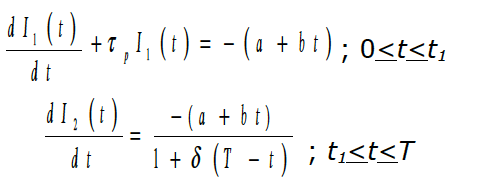(1) (2)

With boundary condition

 I1 (t) =I2 (t) =0 at t=t1 and I1 (t) =IM at t=0

Inventory Level (Q(t))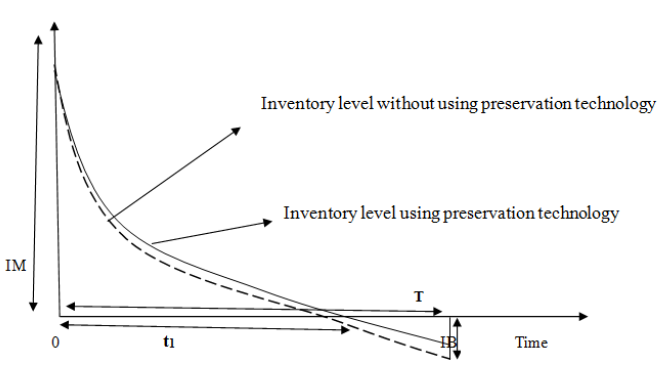Figure 1. Graphical Representation of Inventory System

4. Analytical Solution

Case I: Inventory level without shortage

During the period [0, t1], the inventory depletes due to the deterioration and demand. Hence, the inventory level at any time during [0, t1] is described by differential equation3)

With the boundary condition I1 (t1) = 0 at t=t1

The solution of equation (3) is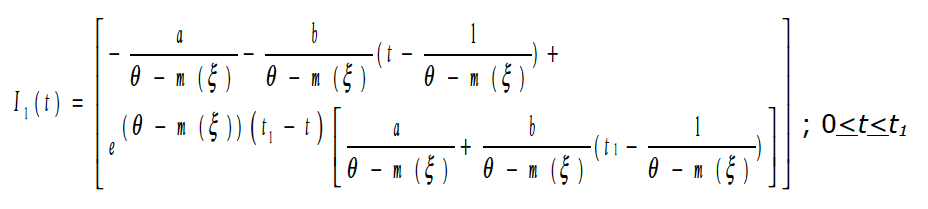(4)

Case II: Inventory level with shortage

During the interval [t1,T] the inventory level depends on demand and a fraction of demand is backlogged. The state of inventory during [t1 ,T] can be represented by the differential equation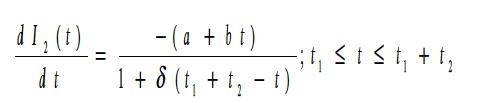(5)

With the boundary condition I2 (t1) = 0 at t=t1

The Solution of equation (5) is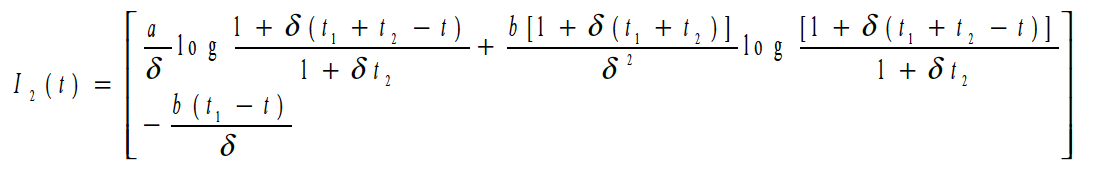(6)

Therefore the total cost per replenishment cycle consists of the following components:

1) Inventory holding cost per cycle;(7)

2) Backordered cost per cycle;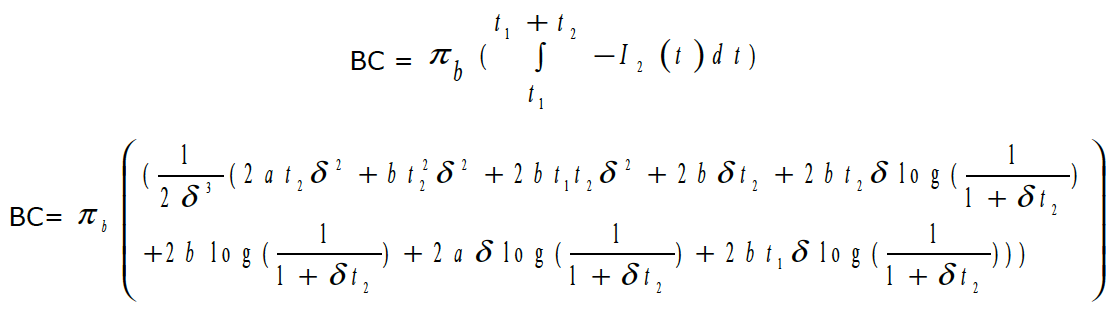(8)

3) Lost sales cost per cycle;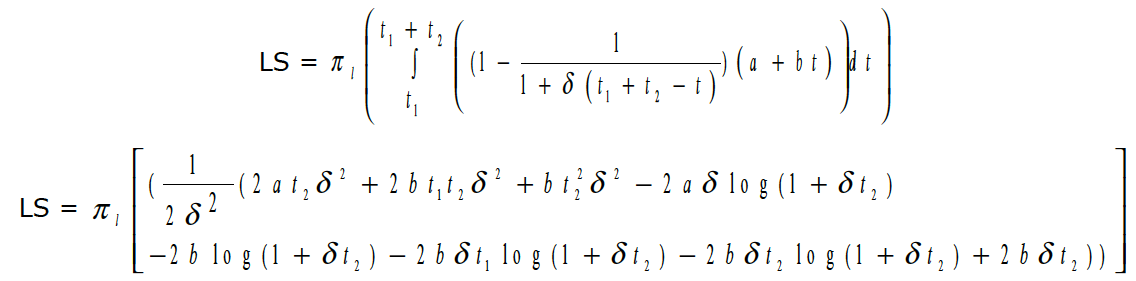(9)

4) Purchase cost per cycle = (purchase cost per unit) * (Order quantity in one cycle)

 PC = C*Q

When t = 0 the level of inventory is maximum and it is denoted by IM (= I1 (0)) then from the equation (4)(10)

The maximum backordered inventory is obtained at t = t1 +t2 then from the equation (6)

 IB = -I2 (t1+t2)(11)

Thus the order size during total time interval [0,T]

 Q = IM + IB

Now from equations (10) and (11)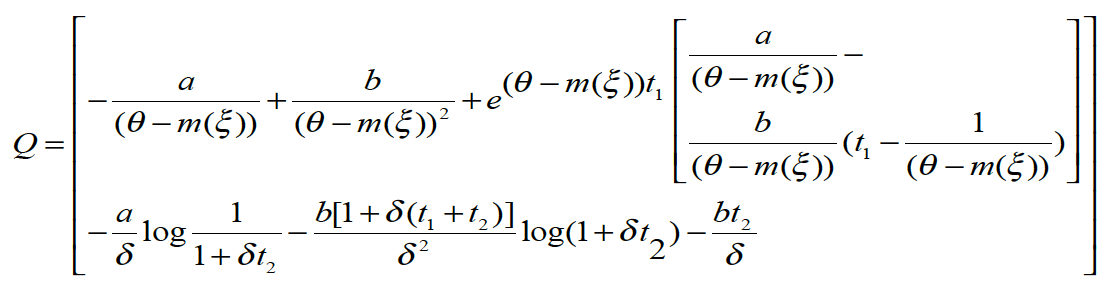(12)

Thus(13)

5) Ordering Cost

 OC = A (14)

Therefore the total cost per time unit is given by,

=[Ordering cost + carrying cost + backordering cost + lost sale cost + purchase Cost]Putting the values of OC, IHC, BC, LS and PC then,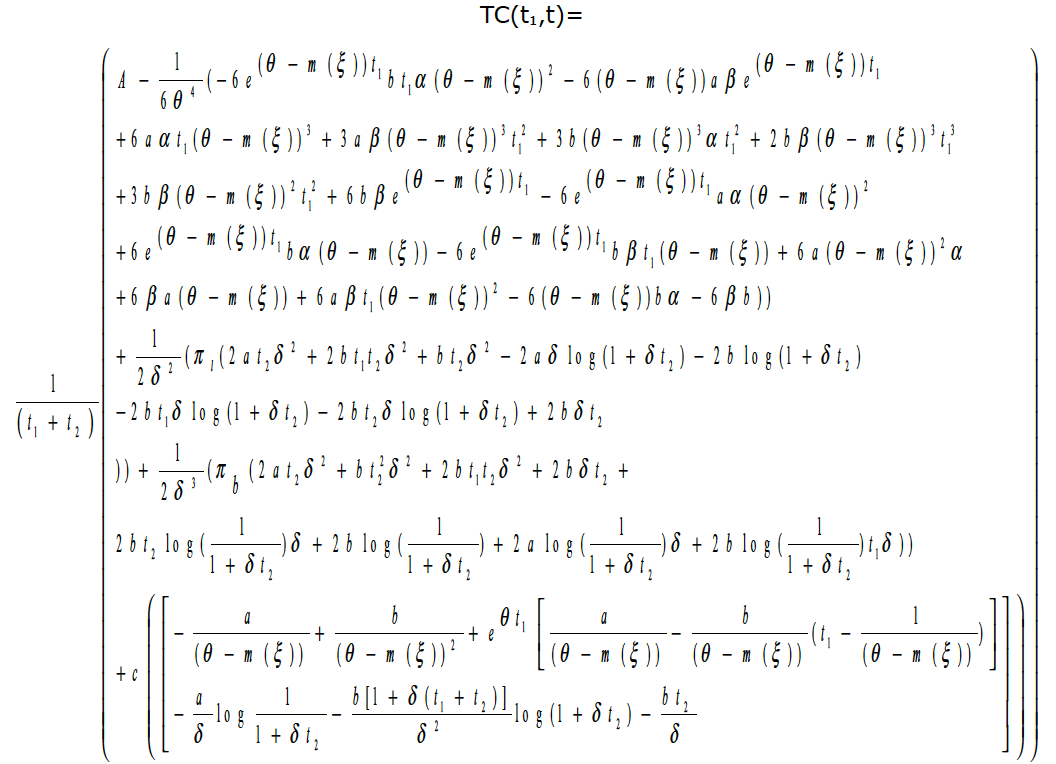(15)

Differentiates the equations (15) with respect to t1, t2 and ξ then we get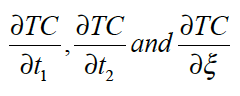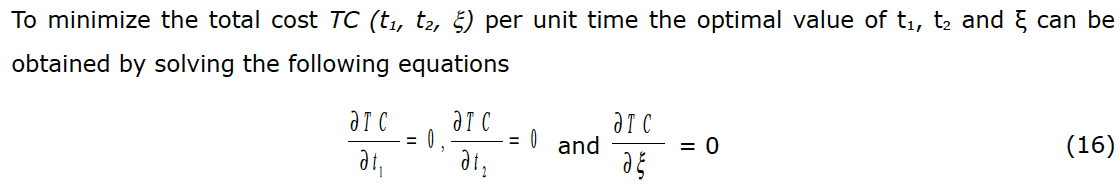Provided the determinant of principal minor of hessian matrix (H-matrix) o TC (t1, t2, x)f is positive definite. i.e. det(H1)>0, det(H2)>0,det(H3)>0 where H1, H2, H3 is the principal minor of the H-matrix.

The H-matrix of function TC (t1, t2, x) is defined as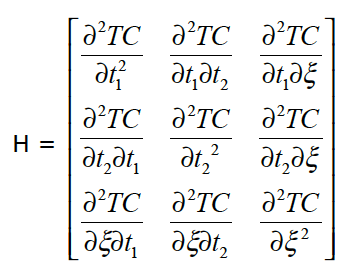5. Numerical Illustration

For numerical illustration we consider an inventory system with the following parameter in proper unit A=250, α=0.5, β=0.01, C=40, πb=10, π1=8, δ=8, a=10, b=2, θ=0.002 and m (ξ)=θ (1-e-2k). The computer output of the program by using maple mathematical software is t1=0.09, t2=1.323 and ξ=9.84. i.e. the value of t1 at which the inventory level become zero is 0.09 unit time, shortage period is 1.323 unit time and the optimal value of preservation technology cost is 9.84 per unit.

6. Conclusion

The purpose of this study is to present an inventory model involving controllable deterioration rate to extend the traditional EOQ model. The products with high deterioration rate are always crucible to the retailer’s business. In real markets, the retailer can reduce the deterioration rate of product by making effective capital investment in storehouse equipment.. In this study to reduce the deterioration rate retailer invested in the PT cost and a solution procedure has presented to determine an optimal replenishment cycle, shortage period, order quantity and preservation technology cost such that the total inventory cost per unit time has minimized. A numerical example has presented to illustrate the model. The model is very practical for the retailers who use the preservation technology in their warehouses to control the deterioration rate under other assumptions of this model. This model can further be extended by taking more realistic assumptions such as finite replenishment rate, Probabilistic demand rate etc.

Acknowledgement

The author would like to thank the editor and anonymous reviewers for their valuable and constructive comments, which have led to a significant improvement in the revised version of the paper.

References

Abad, P.L. (1996). Optimal pricing and lot-sizing under conditions of perishability and partial backordering. Management Science, 42, 1093-1104. http://dx.doi.org/10.1287/mnsc.42.8.1093

Abad, P.L. (2001). Optimal price and order-size for a reseller under partial backlogging. Computers and Operation Research, 28, 53-65. http://dx.doi.org/10.1016/S0305-0548(99)00086-6

Alamri, A.A., & Balkhi, Z.T. (2007). The effects of learning and forgetting on the optimal production lot size for deteriorating items with time varying demand and deterioration rates. International Journal of Production Economics, 107, 125-138. http://dx.doi.org/10.1016/j.ijpe.2006.08.004

Chang, C.T., Teng, J.T., & Goyal, S.K. (2010). Optimal Replenishment policies for non instantaneous deteriorating items. International Journal of Production Economics, 123(1), 62‑68. http://dx.doi.org/10.1016/j.ijpe.2009.06.042

Dave, U., & Patel, L.K. (1981). (T, Si) - policy inventory model for deteriorating items with time proportional demand. Journal of Operational Research Society, 32, 137-142.

Dye, C.-Y., & Ouyang, L.-Y. (2011). A particle swarm optimization for solving joint pricing and lot-sizing problem with fluctuating demand and trade credit financing. Computers & Industrial Engineering, 60(1), 127-137. http://dx.doi.org/10.1016/j.cie.2010.10.010

Dye, C.-Y., Ouyang, L.-Y., & Hsieh, T.-P. (2007). Deterministic inventory model for deteriorating items with capacity constraint and time-proportional backlogging rate. European Journal of Operational Research, 178(3), 789-807. http://dx.doi.org/10.1016/j.ejor.2006.02.024

Ghare, P.M., & Schrader, G.F. (1963). A model for an exponentially decaying inventory. Journal of Industrial Engineering, 14, 238-243.

Goyal, S.K., & Giri, B.C. (2001). Recent trends in modeling of deteriorating inventory. European Journal of Operational Research, 134, 1-16. http://dx.doi.org/10.1016/S0377-2217(00)00248-4

He, Y., Wang, S.-Y., & Lai, K.K. (2010). An optimal production-inventory model for deteriorating items with multiple-market demand. European Journal of Operational Research, 203(3), 593‑600. http://dx.doi.org/10.1016/j.ejor.2009.09.003

Hsu, P.H., Wee H.M., & Teng, H.M. (2010). Preservation technology investment for deteriorating inventory. International Journal of Production Economics, 124(2), 388-394. http://dx.doi.org/10.1016/j.ijpe.2009.11.034

Hung, K.-C. (2011). An inventory model with generalized type demand, deterioration and backorder rates. European Journal of Operational Research, 208(3), 239-242. http://dx.doi.org/10.1016/j.ejor.2010.08.026

Leea Y.-P., & Dye, C.-Y. (2012). An inventory model for deteriorating items under stock-dependent demand and controllable deterioration rate. Computers & Industrial Engineering, 63(2), 474-482. http://dx.doi.org/10.1016/j.cie.2012.04.006

Maihami, R., & Kamalabadi, I.N. (2012). Joint pricing and inventory control for non-instantaneous deteriorating items with partial backlogging and time and price dependent demand. International Journal of Production Economics, 136(1), 116-122. http://dx.doi.org/10.1016/j.ijpe.2011.09.020

Mandal, B. (2010). An EOQ inventory model for Weibull distributed deteriorating items under ramp type demand and shortages. Opsearch, 47(2), 158-165. http://dx.doi.org/10.1007/s12597-010-0018-x

Mishra, V.K,. & Singh, L.S. (2011). Deteriorating inventory model for time dependent demand and holding cost with partial backlogging. International Journal of Management Science and Engineering Management, 6(4), 267-271.

Mishra, V.K., & Singh, L.S. (2010). Deteriorating inventory model with time dependent demand and partial backlogging. Applied Mathematical Sciences, 4(72), 3611-3619.

Ouyang, L.-Y., Wu, K.-S., & Cheng, M.-C. (2005). An inventory model for deteriorating items with exponential declining demand and partial backlogging. Yugoslav Journal of Operations Research, 15(2), 277-288. http://dx.doi.org/10.2298/YJOR0502277O

Roy, A, (2008). An inventory model for deteriorating items with price dependent demand and time varying holding cost. Advanced Modeling and Optimization, 10, 25-37

Skouri, K., Konstantaras, I., Papachristos, S., & Ganas, I. (2009). Inventory models with ramp type demand rate, partial backlogging and Weibull deterioration rate. European Journal of Operational Research, 192, 79-92. http://dx.doi.org/10.1016/j.ejor.2007.09.003

Whitin, T.M. (1957). The Theory of Inventory Management. 2nd ed. Princeton, NJ: Princeton University Press.